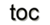Next: 1.8 Overview of Theoretical Up: 1. Mathematical Concepts Previous: 1.6 Fiber Bundles and

# 1.7 Discrete Electromagnetics

Several issues related to the differential formulation of the Maxwell equations were presented in Section 1.2. Finally, this section introduces a concise way of formulating physical problems regarding the fiber bundle and algebraic topology concepts. Starting with the integral formulation and partially reinserting the geometrical objects expressed in vector calculus notation, but omitting the orientation reads: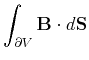(1.51)(1.52)

A better-suited representation, which directly references the oriented geometric object a quantity is assigned to, is given with the formalism of ordinary and twisted differential forms which can be seen as the continuous counterpart of cochains, as introduced in Section 1.4. For a brief introduction of this topic, a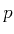-dimensional differential form, or short-form, can be seen as the subject to integration on-dimensional domains [60,24]. If the domain is internally oriented, then the-form is called ordinary-form which is denoted by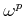and the corresponding externally oriented-form is called twisted, denoted by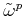. By the concept of a multivector (see Section 1.4), a-form is given as a linear function on the space of multivectors with values in an algebraic field. Then it follows that the pairing of a multivector, or-vector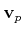, and a-formgives a value like the pairing of a chain and cochain [61,35], as given in Section 1.4.4. This analogy suggests the following representation of the pairing of a-vector and a-form: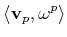(1.53)

The duality property, stated by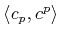, between chains and cochains transfers directly to the continuous multivectors and-forms, introduced in Section 1.5. This is an important step towards a formal, consistent, and computationally manageable concept. A-formon a continuous domaincan then be correlated to its discrete counterpart on a cell complex, a cochain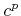, since it associates a value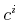with each cell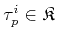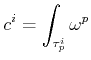(1.54)

Another correspondence between cochains and-forms is given by the concept of the coboundary operator. As introduced in Section 1.4.2, the coboundary operator is defined to allow the transition from a topological equation of the form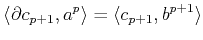(1.55)

to the following relation between cochains: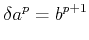(1.56)

The continuous differential forms can then be related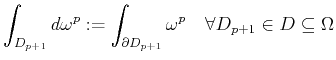(1.57)

where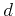is an operator transforming-forms into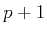-forms. This operator is called the exterior differential and mimics the property of the coboundary operator by transforming a topological equation given in integral form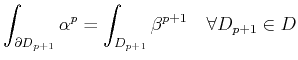(1.58)

into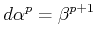(1.59)

Given the properties of the coboundary operator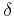, the exterior differentialcan be seen as the continuous counterpart  of. The following table depicts the correspondence between discrete and continuous concepts .

 discrete setting continuous setting-cell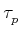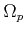-dimensional domain boundary of a-cell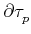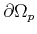boundary of a-dimensional domain-chain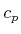weighted-domain-cochain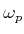-differential form pairing of-chain and-cochain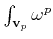weighted-integral of a-form coboundary operatorexterior differential operator

Based on these concepts, the local vector field representation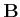becomes an ordinary 2-formand the scalar field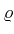a twisted 3-form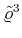: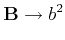(1.60)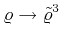(1.61)

The numbers and orientation give the dimension on which these quantities are to be integrated. The adjoint of the exterior differential as the boundary of a weighted domain is the generalized Stoke's theorem: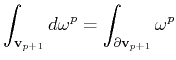(1.62)

It has to be noted, that the given differential form expression is more general than the vector calculus notation due to the fact that the expression is valid for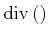,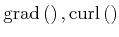and the discrete chain and cochain representations automatically express the type of the dimension with the general notion of: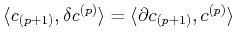(1.63)

Examples of-form complexes for differential operators encountered in different works [62,24] for vector analysis in three dimensions are denoted by: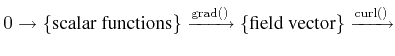(1.64)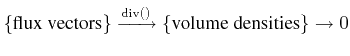The concept of constitutive links closes the gap between ordinary and twisted cochains with discrete links between them. Two different types can be obtained: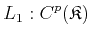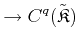(1.65)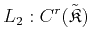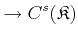(1.66)

The inherently discrete computer implementation can now be equipped with all the necessary information and structure regarding the physical entities.Next: 1.8 Overview of Theoretical Up: 1. Mathematical Concepts Previous: 1.6 Fiber Bundles and

R. Heinzl: Concepts for Scientific Computing